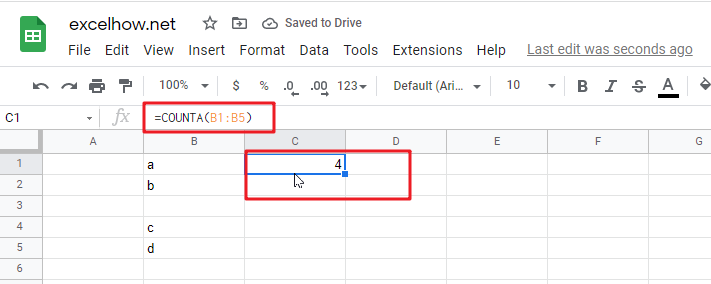# ExcelHow

This post will guide you how to use Google Sheets COUNTA function with syntax and examples in Google Spreadsheet.

## Description

The Google Sheets COUNTA function counts the number of cells that are not empty in a range. And it returns the number of non-blank cells within a range or values. It can be used to count all the cells in a range that contain numbers, text, logical values, error values and empty text string.

The COUNTA function is a build-in function in Google Spreadsheet and it is categorized as a Statistical Function.

## Syntax

The syntax of the COUNTA function is as below:

`= COUNTA(value1, [value2],…)`

Where the COUNTA function arguments are:

• `value1 `-This is a required argument.  The first item, cell reference, or range within which you want to count numbers.
• `Value2 `– This is an optional argument. You can enter up to 255 value arguments.

Note:

• The COUNTA function does not count empty cells.
• If you want to only count cells that contain numbers, just use the COUNT function.
• If you want to count logical values, text, or error values, you can use the COUNTA function.

### Google Sheets COUNTA Function Examples

The below examples will show you how to use Google Sheets COUNTA Function to count the number of cells in a range that are not empty.

#1 To count the number of cells in the range B1:B4, just using the following excel formula:

`=COUNTA(B1:B4)`